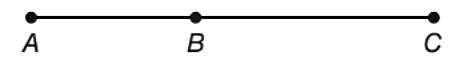Chapter P.3, Problem 36EElementary Geometry For College St...

7th Edition
Alexander + 2 others
ISBN: 9781337614085

Solutions

Chapter
SectionElementary Geometry For College St...

7th Edition
Alexander + 2 others
ISBN: 9781337614085
Textbook Problem

In Exercises 35 to 38, with A - B - C on A C ¯ , it follows that A B + B C = A C .Find A B if A C = 25 and B C = 11 .

To determine

To find:

AB for AC=25 and BC=11.

Explanation

Calculation:

Given,

AC=25

BC=11.

AB+BC=AC.

Substitute; AC=25 and BC=11 in the above relation,

AB+11=25

Add; -11 on both sides,

Still sussing out bartleby?

Check out a sample textbook solution.

See a sample solution

The Solution to Your Study Problems

Bartleby provides explanations to thousands of textbook problems written by our experts, many with advanced degrees!

Get Started

Evaluate limx0sin(3+x)2sin9x.

Calculus (MindTap Course List)

Find the median for the following set of scores: 1, 9, 3, 6, 4, 3, 11, 10

Essentials of Statistics for The Behavioral Sciences (MindTap Course List)

Evaluate the definite integrals in Problems 1-32. 27.

Mathematical Applications for the Management, Life, and Social Sciences